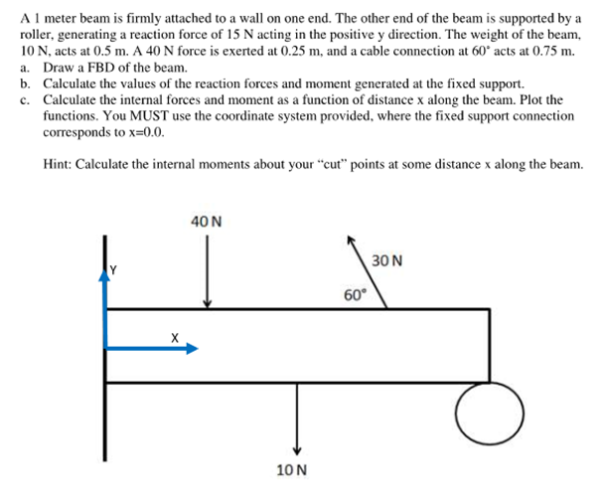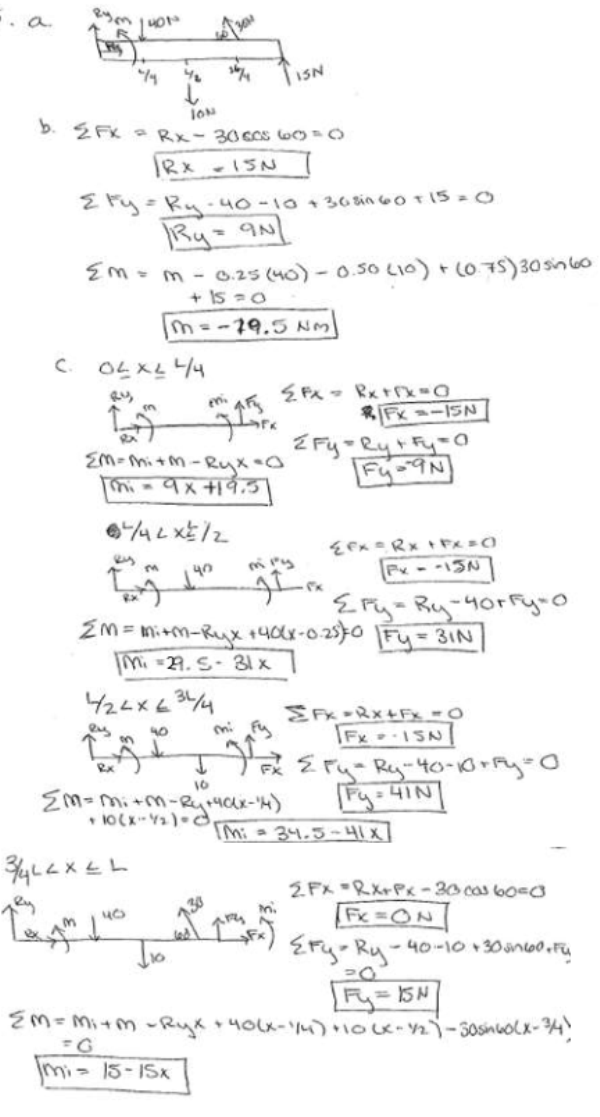A 1 meter beam is firmly attached to a wall on one end. The other end of the beam is supported by a roller, generating a reaction force of 15 N acting in the positive y direction. the wight of the beam, 10 N, acts at 0.5 m. A 40 N force is exerted at 0.25 m, and a cable connection at 60 degrees acts at 0.75 m. a) Draw a FBD of the beam. b) Calculate the values of the reaction forces and moment generated at the fixed support. c) Calculate the internal forces and moment as a function of distance x along the beam. Plot the functions. You must use the coordinate system provided, where the fixed support connection corresponds to x=0.0.A 1 meter beam is firmly attached to a wall on one end. The other end of the beam is supported by a roller, generating a reaction force of 15 N acting in the positive y direction. the wight of the beam, 10 N, acts at 0.5 m. A 40 N force is exerted at 0.25 m, and a cable connection at 60 degrees acts at 0.75 m. a) Draw a FBD of the beam. b) Calculate the values of the reaction forces and moment generated at the fixed support. c) Calculate the internal forces and moment as a function of distance x along the beam. Plot the functions. You must use the coordinate system provided, where the fixed support connection corresponds to x=0.0.

biomechanics page 1 biomechanics biomechanics biomechanics biomechanics biomechanics biomechanics biomechanics biomechanics page 2